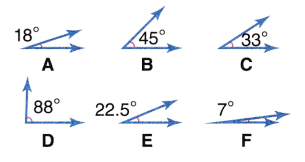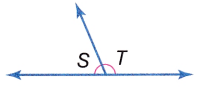# McGraw Hill Math Grade 6 Lesson 22.1 Answer Key Measuring Angles

Practice questions available in McGraw Hill Math Grade 6 Answer Key PDF Lesson 22.1 Measuring Angles will engage students and is a great way of informal assessment.

Exercises

IDENTIFY

Question 1.
Is the angle acute or obtuse?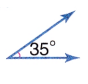Explanation:
An angle which is measuring less than 90 degrees is called an acute angle. This angle is smaller than the right angle so the given figure is acute angle.

Question 2.
Is the angle acute or obtuse?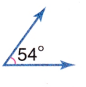Explanation:
An angle which is measuring less than 90 degrees is called an acute angle. This angle is smaller than the right angle so the given figure is an acute angle.

Question 3.
Is the angle acute, obtuse, or right?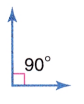Explanation:
The above angle is exactly equal to 90 degrees. So, it is the right angle.

Question 4.
Is the angle acute, right, or obtuse?Explanation:
An angle that is measuring greater than 90 degrees is called an obtuse angle. This angle is greater than the right angle so the given figure is an obtuse angle.

Question 5.
Is the angle acute, right, or obtuse?Explanation:
An angle which is measuring less than 90 degrees is called an acute angle. This angle is smaller than the right angle so the given figure is an acute angle.

Question 6.
How many degrees do you need to subtract from the angle to make it acute?To make the angle acute we have to subtract 90 from 130.
130 – 90 = 40

Question 7.
How many more degrees would you have to add to the angle to make it an obtuse angle?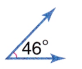46 + 45 = 91
By adding more than 45 degrees we can get the obtuse angle.

Question 8.
Can you subtract one obtuse angle from another and still have an obtuse angle?
We cannot get an obtuse angle by subtracting one obtuse angle from another.
Let us say one angle is 110 and the other angle is 100
110 – 100 = 10 (cannot be obtuse angle)

Question 9.
What three angles below could you add together and not get an obtuse angle?